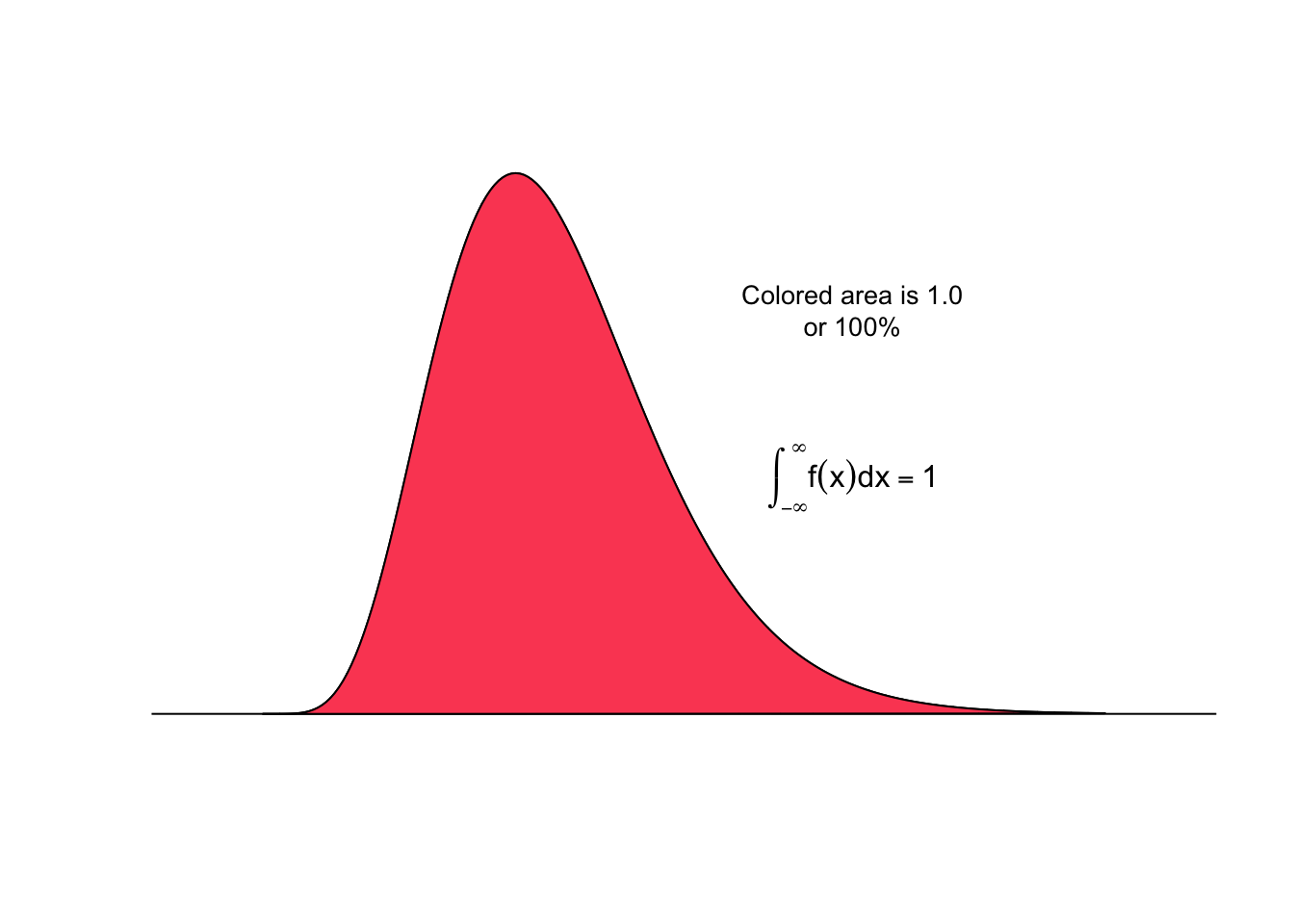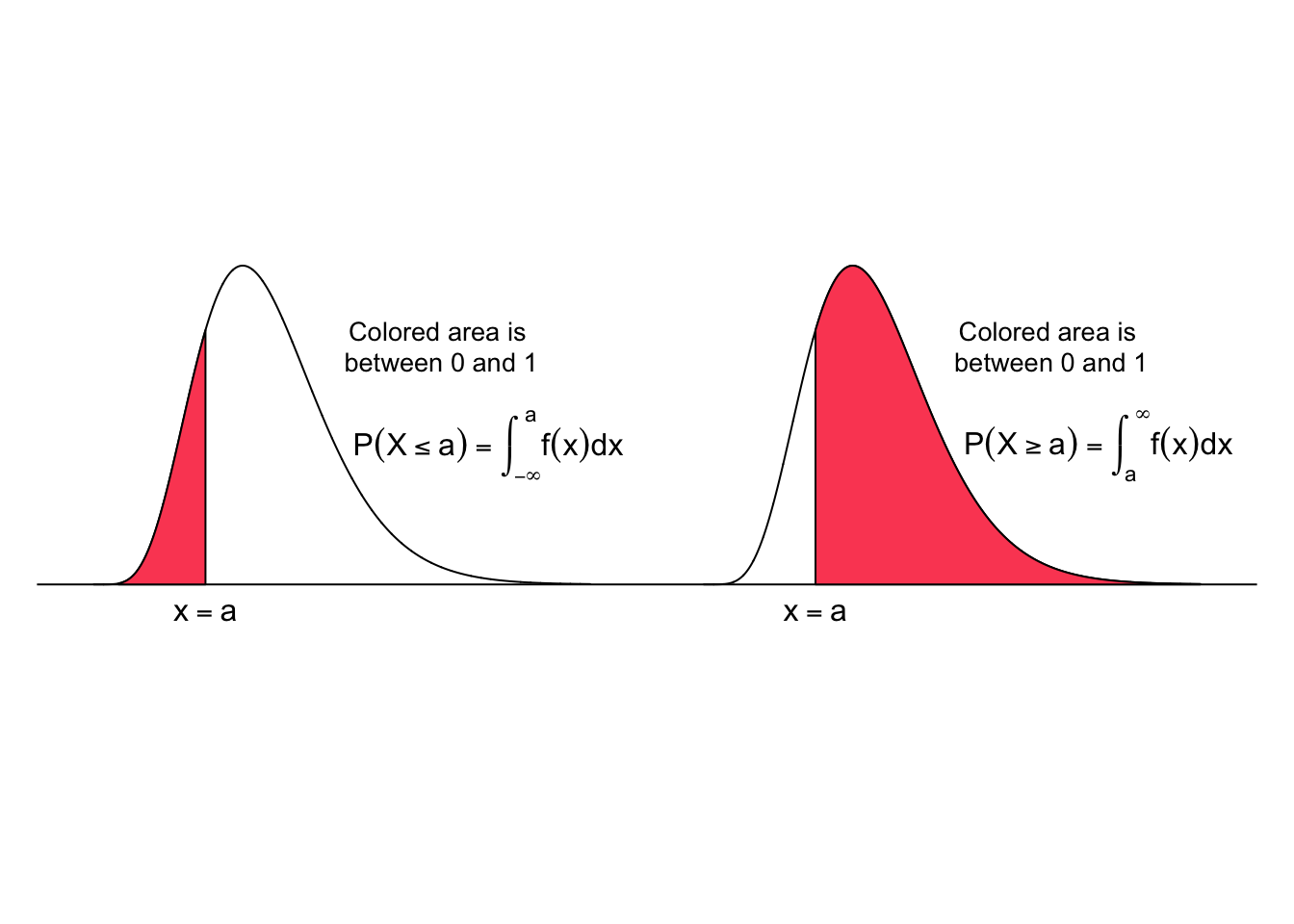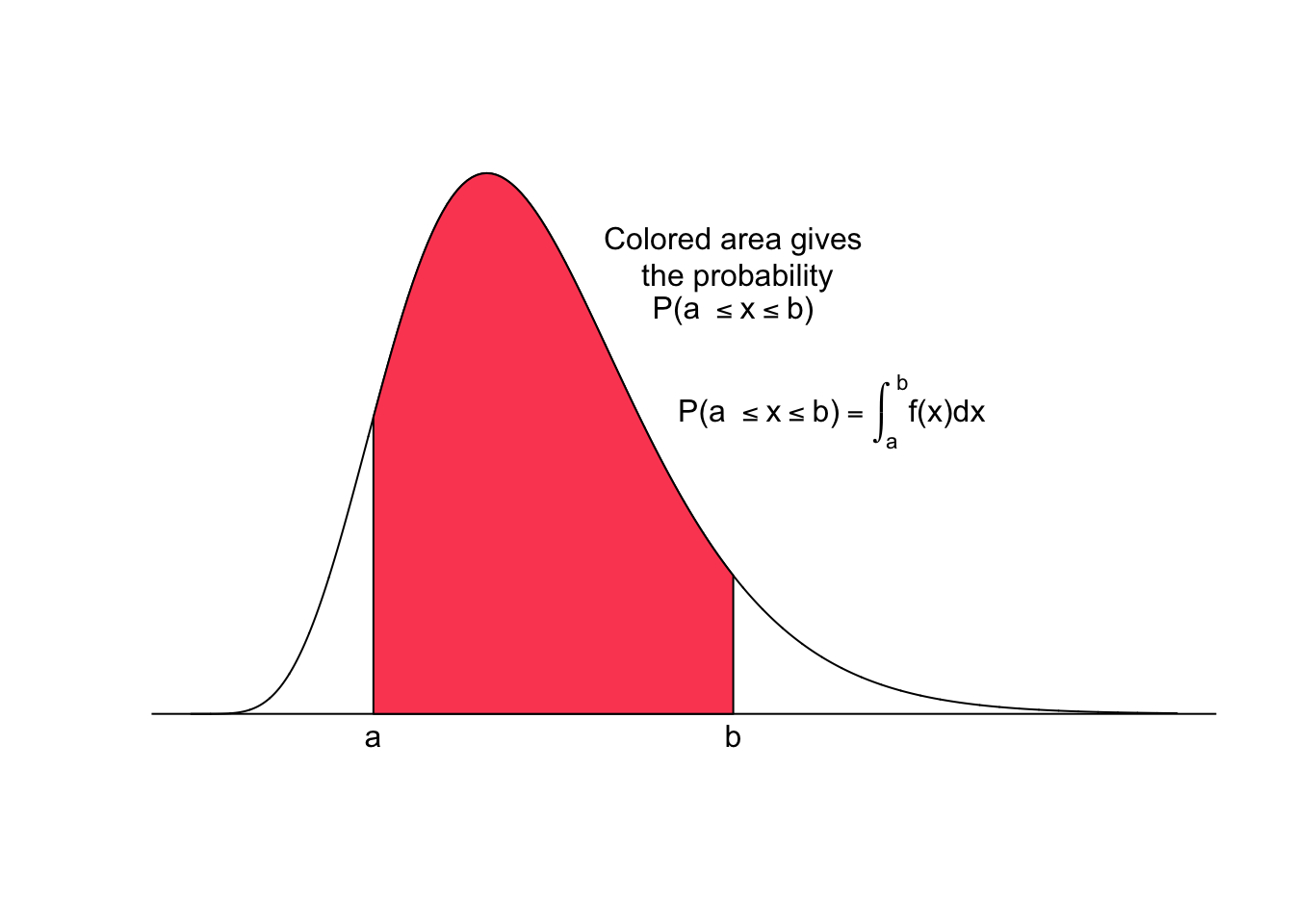The shape of the distribution of a random variable may be visualized with a smooth curve. Such curves, which represent the distribution of continuous variables, are called probability density functions (PDF) or just density functions. Probability density functions have three main properties (Mann 2012, Weiss 2010):

1. A PDF plots always on or above the horizontal axis, and
2. the total area under a PDF (and above the horizontal axis) equals 1, and thus any value in any interval lies in the range 0 to 1, and
3. all possible observations of the variable that lie within any specified range equals the corresponding area under the density function and can be expressed as percentages or proportions.

The area under the curve is computed by the integral of the value $$x$$ from $$-\infty$$ to $$+\infty$$, which yields 1. $\int_{-\infty}^{+\infty} f(x)dx = 1$The probability that a continuous random variable $$x$$ takes a value within a certain interval is given by the area under the curve between the two limits of the interval. The colored area under the curve for the interval $$(-\infty, a]$$ (left panel) and for the interval $$[a,\infty)$$ (right panel) is shown in the figure below.

The probability that $$x$$ falls in the interval $$(-\infty, a]$$ is

$P(X \le a) = \int_{-\infty}^{a}f(x)dx$

and the probability that $$x$$ falls in the interval $$[a, \infty)$$ is

$P(X \ge a) = 1 - P(X \le a) = \int_{a}^{\infty}f(x)dx$The probability that a continuous random variable $$x$$ assumes a value within a certain interval is given by the area under the curve between the two limits of the interval. The colored area under the curve from $$a$$ to $$b$$ in the figure below gives the probability that $$x$$ falls in the interval $$[a,b]$$.\begin{align} P(a \le x \le b) & = \int_{a}^{b}f(x)dx\\ & = P(x \le b) - P(x \le a) \\ & = \int_{-\infty}^{b}f(x)dx - \int_{-\infty}^{a}f(x)dx \end{align}

Note that the interval $$a \le x \le b$$ states that $$x$$ is greater than or equal to $$a$$ but less than or equal to $$b$$.

For a continuous probability distribution, the probability is always calculated for an interval. The probability that a continuous random variable $$x$$ assumes a single value is always zero. This is because, the probability to pick exactly one value out of an infinite number of value $$\in \mathbb R$$ is zero. In a geometric sense this means that the area of a line, which represents a single point, is zero.

$P(x) = 0$

From this we can deduce that for a continuous random variable

$P(a \le x \le b) = P(a < x < b)$

In other words, the probability that $$x$$ assumes a value in the interval $$a$$ to $$b$$ is the same whether or not the values $$a$$ and $$b$$ are included in the interval.